# AP Physics 1 Multiple-Choice Practice Test 36

### Test Information10 questions18 minutes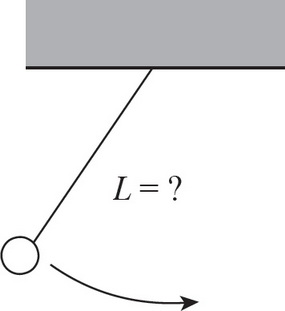1. A simple pendulum oscillates back and forth with a period of 2 s. What is the length of the string of the pendulum?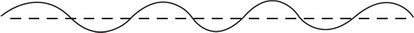2. The speed of sound in air is 343 m/s. If the wavelength of a certain sound wave is 20.0 cm, what is its frequency?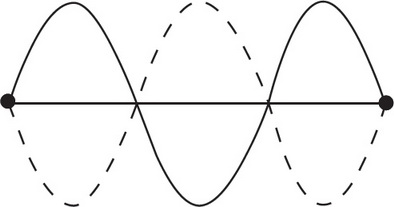3. Which of the following is a true statement about standing waves?4. A string of length 2 m that's fixed at both ends supports a standing wave with a wavelength of 0.8 m. What is the harmonic number of this standing wave?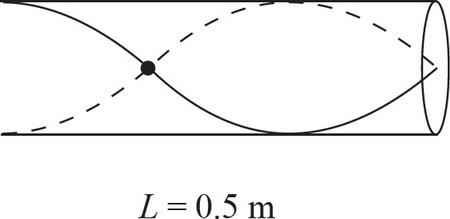5. What is the frequency of the third harmonic standing wave in an open tube with a length of 0.5 m? The speed of sound in the tube is 343 m/s.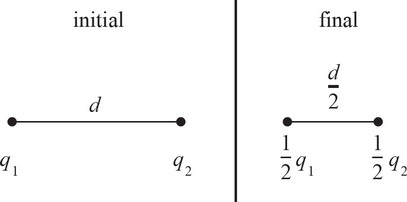6. What happens to the magnitude of the electric force between two opposite charges if the magnitude of each charge is halved and the distance between their centers is halved?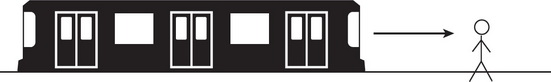7. As a train approaches the next stop, the conductor blows the horn and applies the brakes on the train. What will a person standing at the train stop hear as the train approaches?

8. A speaker is emitting a sound wave with a wavelength λ. If a person is standing in an adjacent room, which of the following would NOT affect the perceived wavelength of the wave?

9. Question below refers to the circuit below: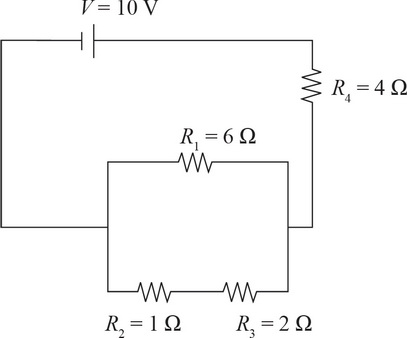What is the total resistance of the circuit?

10. Question below refers to the circuit below:How long will it take for the battery to deliver 300 J of energy to the circuit?